## Example Questions

### Example Question #1 : Mixed / Improper Fractions

Write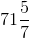as an improper fraction.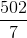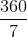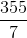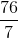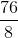Explanation:

To find the improper fraction value, we must effectively add together 71 and 5/7. To do this, we will give 71 a denominator of 7; therefore, we are transforming 71/1 to x/7.  The shortest way to do this is to multiply by 7/7 (which really is 1); therefore, 71 = 71 * (7/7) = 497/7.

Now add them: (497 + 5)/7 = 502/7

### Example Question #2 : Mixed / Improper Fractions

Which of the following improper fractions is equivalent to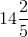?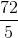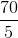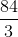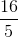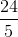Explanation:

To find an improper fraction, you need to multiply the whole number that you have by the denominator of the associated fraction.  For our problem, this means that you will multiplyby, getting.  Next, you add this to the numerator of your fraction, giving you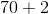, or.  Finally, you place this over your original denominator, giving you:### Example Question #3 : How To Find Out An Improper Fraction From A Mixed Fraction

Which of the following improper fractions is equivalent to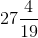?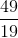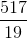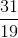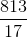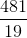To find an improper fraction, you need to multiply the whole number that you have by the denominator of the associated fraction.  For our problem, this means that you will multiplyby, getting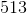.  Next, you add this to the numerator of your fraction, giving you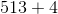, or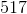.  Finally, you place this over your original denominator, giving you: